Domain

Definition

General definition

The domain of a function is the set of inputs allowed for the function, i.e., the set of values that can be fed into the function to give a valid output.

If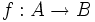$f:A \to B$ is a function, the domain of$f$ is the set$A$.

For a function described by an expression or procedure without explicit domain specification

If a function of one variable (i.e., a function whose domain is a subset of the reals) is defined by means of an expression or procedure, but the domain is not explicitly specified, the domain is taken to be the maximum possible domain, i.e., the largest subset of the reals on which that expression or procedure makes sense and yields a meaningful answer.

On the other hand, the domain of the function may be specified explicitly to be a proper subset of the maximum possible domain that we could infer from the expression alone.

Caveats

Domain and restriction of domain

The study of a function depends crucially on the domain on which the function is being studied. If a function of one variable is defined solely by means of an expression or procedure, the domain of the function is taken to be the largest possible subset of the reals on which that expression or procedure makes sense and gives a valid answer. However, we can also consider functions restricted to domains that are strictly smaller than the maximum possible domain on which the expression being used for the function makes sense. The behavior of the function, as well as answers to questions like whether it is increasing or decreasing and what its extreme values are, depends on what domain we are considering the function on.

Here are some examples:

• Consider the function$f$ defined as follows: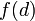$f(d)$ is defined as the area of a circle with diameter$d$. Ignoring the boundary case of point circles and line circles, the only possible inputs for this function are positive reals, so$f$ is a function from the positive reals to the positive reals given by the expression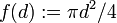$f(d) := \pi d^2/4$. However, if we look only at the expression for$f$, then that expression makes sense for all real numbers, including zero and negative real numbers as well as positive real numbers. Call the latter function$g$, i.e.,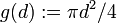$g(d) := \pi d^2/4$ for all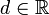$d \in \R$. Then,$f$ is the restriction of$g$ to the subdomain$(0,\infty)$. Note that:
•$g$ is not an increasing function, but the restriction$f$ is an increasing function.
•$g$ is not a one-one function, but the restriction$f$ is a one-one function.
•$g$ attains its absolute minimum value, but the restriction$f$ does not.

Computation of domain

Computation of maximum possible domain from algebraic expression

We discuss below how we can compute the domain of a function obtained by using a pointwise combination, composite, inverse function, and piecewise definition:

Method for constructing new functions from old In symbols Domain of the new function in terms of domains of the old functions Additional notes
pointwise sum$f + g$ is the function$x \mapsto f(x) + g(x)$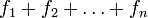$f_1 + f_2 + \dots + f_n$ is the function$x \mapsto f_1(x) + f_2(x) + \dots + f_n(x)$
pointwise difference$f - g$ is the function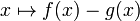$x \mapsto f(x) - g(x)$ Intersection of the domains of the functions being subtracted Similar to the note for sums
scalar multiple by a constant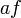$af$ is the function$x \mapsto af(x)$ where$a$ is a real number Same as the domain of the original function
pointwise product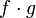$f \cdot g$ (sometimes denoted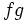$fg$) is the function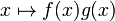$x \mapsto f(x)g(x)$
f_1 \cdot f_2 \cdot \dots f_n[/itex] (sometimes denoted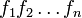$f_1f_2\dots f_n$ is the function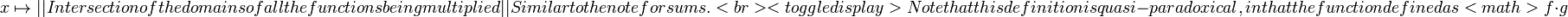$x \mapsto || Intersection of the domains of all the functions being multiplied || Similar to the note for sums.
where$f(x) = x - 2$ and$g(x) = 1/(x - 2)$ is 1 everywhere except at 2. This is because even though the expressions for$f$ and$g$ cancel each other, evaluating at$x = 2$ does not make sense for the expression prior to algebraic simplication by cancellation.</toggledisplay>